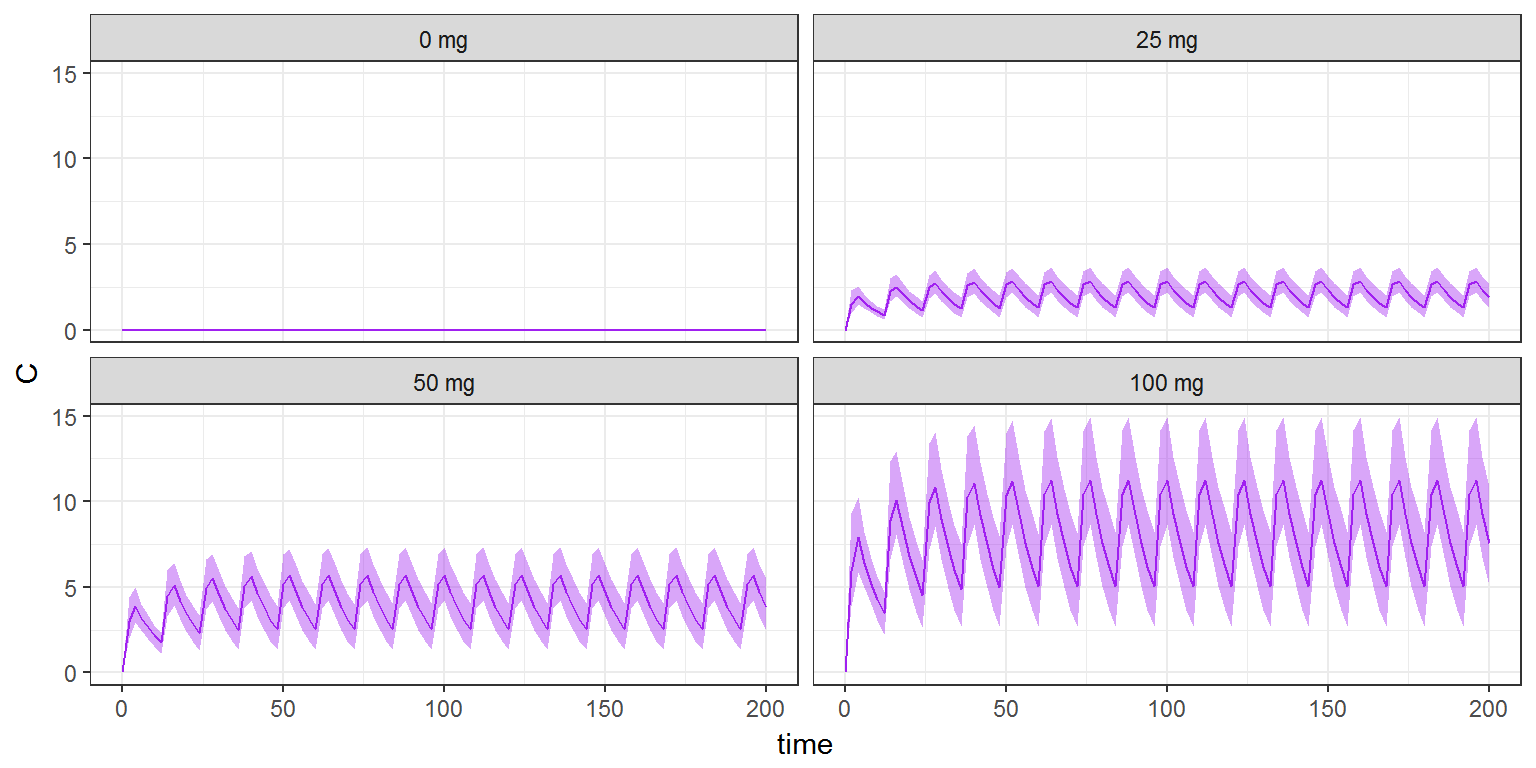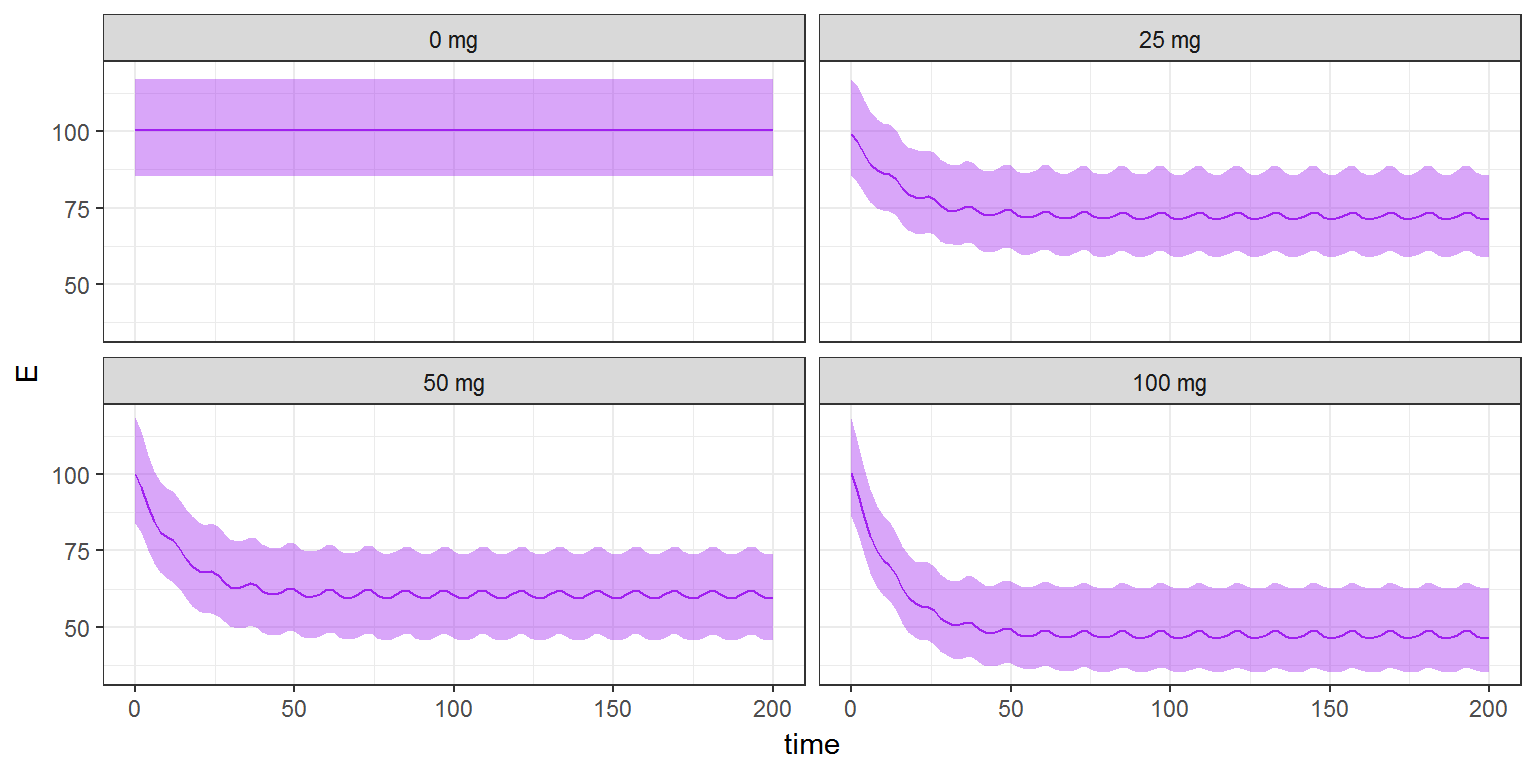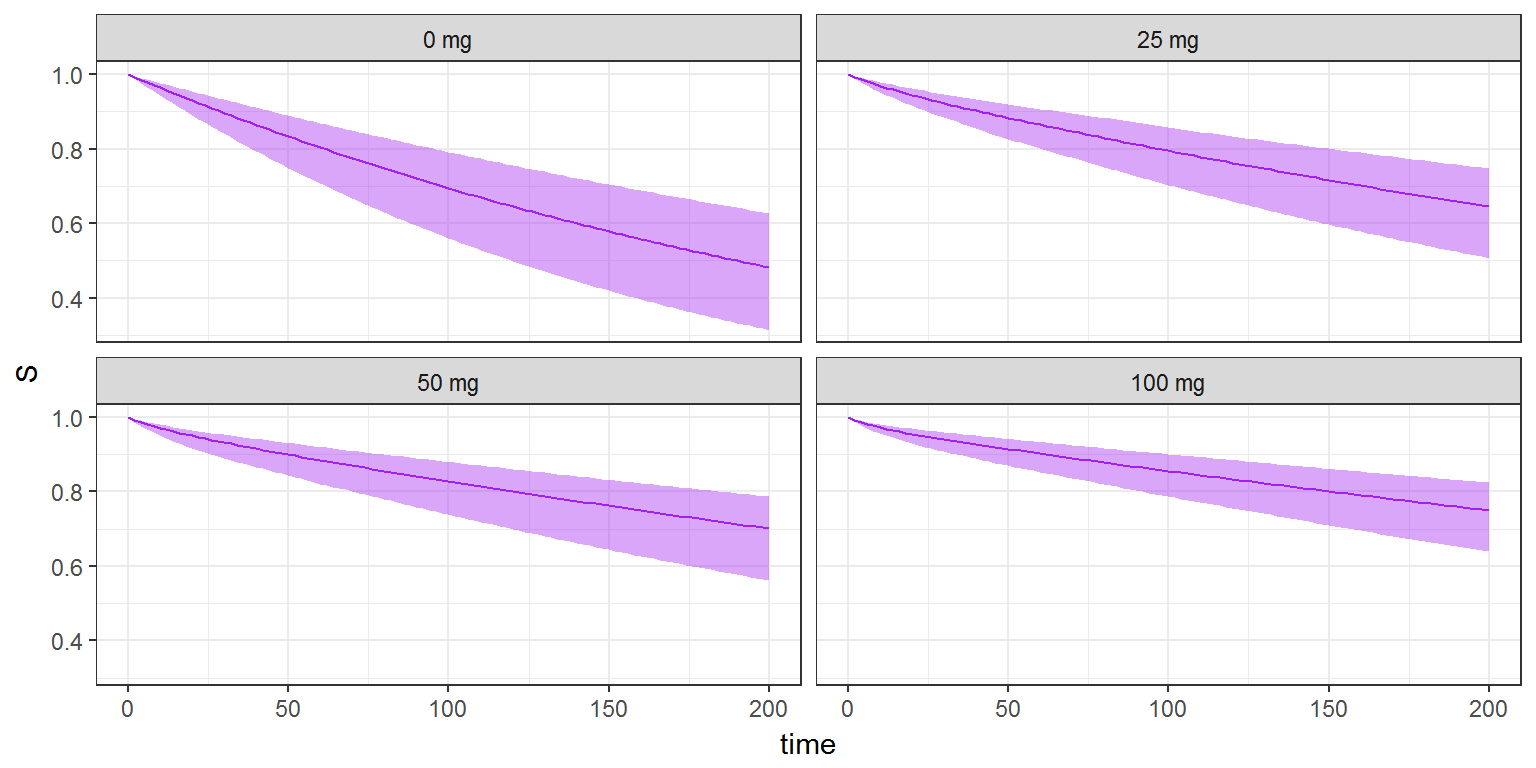$$\newcommand{\esp}{\mathbb{E}\left(#1\right)} \newcommand{\var}{\mbox{Var}\left(#1\right)} \newcommand{\deriv}{\dot{#1}(t)} \newcommand{\prob}{ \mathbb{P}\!(#1)} \newcommand{\eqdef}{\mathop{=}\limits^{\mathrm{def}}} \newcommand{\by}{\boldsymbol{y}} \newcommand{\bc}{\boldsymbol{c}} \newcommand{\bpsi}{\boldsymbol{\psi}} \def\pmacro{\texttt{p}} \def\like{{\cal L}} \def\llike{{\cal LL}} \def\logit{{\rm logit}} \def\probit{{\rm probit}} \def\one{{\rm 1\!I}} \def\iid{\mathop{\sim}_{\rm i.i.d.}} \def\simh0{\mathop{\sim}_{H_0}} \def\df{\texttt{df}} \def\res{e} \def\xomega{x} \newcommand{\argmin}{{\rm arg}\min_{#1}} \newcommand{\argmax}{{\rm arg}\max_{#1}} \newcommand{\Rset}{\mbox{\mathbb{R}}} \def\param{\theta} \def\setparam{\Theta} \def\xnew{x_{\rm new}} \def\fnew{f_{\rm new}} \def\ynew{y_{\rm new}} \def\nnew{n_{\rm new}} \def\enew{e_{\rm new}} \def\Xnew{X_{\rm new}} \def\hfnew{\widehat{\fnew}} \def\degree{m} \def\nbeta{d} \newcommand{\limite}{\mathop{\longrightarrow}\limits_{#1}} \def\ka{k{a}} \def\ska{k{\scriptscriptstyle a}} \def\kel{k{e}} \def\skel{k{\scriptscriptstyle e}} \def\cl{C{\small l}} \def\Tlag{T\hspace{-0.1em}{lag}} \def\sTlag{T\hspace{-0.07em}{\scriptscriptstyle lag}} \def\Tk{T\hspace{-0.1em}{k0}} \def\sTk{T\hspace{-0.07em}{\scriptscriptstyle k0}} \def\thalf{t{1/2}} \newcommand{\Dphi}{\partial_\pphi #1} \def\asigma{a} \def\pphi{\psi} \newcommand{\stheta}{{\theta^\star}} \newcommand{\htheta}{{\widehat{\theta}}}$$

In the second part of this demonstration the between patient variability will be included to run population PKPD simulations. This will provide a more realistic prediction on the outcome among a large number of patients.

# The PKPD model

The same PKPD model as in part I is used. To include the between-patient variablity the section INDIVIDUAL is added. In this section the distribution type and the distribution parameters of the PK parameters are sepcified.

Here log-normal distributions are used, except for $$Imax$$. Since $$Imax$$ can only take values between 0 and 1 a logit-normal distribution is applied.

This new joint PKPD model is implemented in the text file model/cts2II.txt:

[LONGITUDINAL]
input={Tk0,V,Cl,Imax,E0,IC50,kout,alpha,beta}

EQUATION:
C = pkmodel(Tk0, V, Cl)

E_0 = E0
kin = E0*kout
ddt_E = kin*(1-Imax*C/(C+IC50)) - kout*E

h = (alpha/1000)*exp(beta*E)
H_0 = 0
ddt_H = h
S = exp(-H)

[INDIVIDUAL]
input={Tk0_pop,V_pop,Cl_pop,Imax_pop,E0_pop,IC50_pop,kout_pop,alpha_pop,
beta_pop, omega_Tk0,omega_V,omega_Cl,omega_Imax,omega_E0,omega_IC50,
omega_kout,omega_alpha,omega_beta}

DEFINITION:
Tk0   = {distribution=lognormal,   prediction=Tk0_pop,  sd=omega_Tk0}
V     = {distribution=lognormal,   prediction=V_pop,    sd=omega_V}
Cl    = {distribution=lognormal,   prediction=Cl_pop,   sd=omega_Cl}
E0    = {distribution=lognormal,   prediction=E0_pop,   sd=omega_E0}
IC50  = {distribution=lognormal,   prediction=IC50_pop, sd=omega_IC50}
kout  = {distribution=lognormal,   prediction=kout_pop, sd=omega_kout}
Imax  = {distribution=logitnormal, prediction=Imax_pop, sd=omega_Imax}
alpha = {distribution=lognormal,   prediction=alpha_pop,sd=omega_alpha}
beta  = {distribution=lognormal,   prediction=beta_pop, sd=omega_beta}


# Simulating inter individual variability (IIV)

The simulation here with simulx is analogous to the simulation in part I. One difference is that now we specify the number of patient to be used for the simulation. For each patient simulx will take a random sample from the parameter distributions and run a simulation. As a result we will obtain the distributions for the concentration $$C$$, disease process $$E$$ and survival $$S$$. In other words simulx produces by Monte Carlo simulation the predicted distributions of $$C$$, $$E$$ and $$S$$. A large number of patients must then be simulated for each group in order to estimate correctly these distributions. Here, the size of the Monte Carlo is $$N=1000$$ for each group.

The input for simulx to define four groups with four different doses and 1000 patients is:

N=400
g1   <- list(treatment = trt1, size=N, level='individual')
g2   <- list(treatment = trt2, size=N, level='individual')
g3   <- list(treatment = trt3, size=N, level='individual')
g4   <- list(treatment = trt4, size=N, level='individual')

We use the same PKPD population parameters for the four groups.

pop.param   <- c(
Tk0_pop   = 3,    omega_Tk0   = 0.2,
V_pop     = 10,   omega_V     = 0.2,
Cl_pop    = 1,    omega_Cl    = 0.2,
Imax_pop  = 0.8,  omega_Imax  = 0.5,
E0_pop    = 100,  omega_E0    = 0.1,
IC50_pop  = 4,    omega_IC50  = 0.1,
kout_pop  = 0.1,  omega_kout  = 0.1,
alpha_pop = 0.5,  omega_alpha = 0.2,
beta_pop  = 0.02, omega_beta  = 0
)

We specify the output for simulx by defining a list output. Here, this list tells simulx to compute the predicted concentration $$Cc$$, the predicted effect $$E$$ and the predicted survival function $$S$$ at every hour, starting at time 0.

f <- list(name = c('C','E','S'),
time = seq(0,200,by=2))

We can now run simulx with these input arguments:

res <- simulx(model     = "model/cts2II.txt",
parameter = pop.param,
group     = list(g1,g2,g3,g4),
output    = f)

The list res contains the results of all the simulations.

We will use the function prctilemlx.R to compute and display several prediction intervals on a same plot using different shades of colour.

labels <- c("0 mg", "25 mg", "50 mg", "100 mg")
prctilemlx(res$C, number=2, level=90, labels=labels) + theme(legend.position = "none")prctilemlx(res$E, number=2, level=90, labels=labels) + theme(legend.position = "none")prctilemlx(res\$S, number=2, level=90, labels=labels) + theme(legend.position = "none")The simulations in this demonstration gave us the distribution of the concentration, disease progression and survival. However, in reality, where only a finite number of patients are included, this can not be observed. Therefore, in the next step to have more realistic simulations, a small number of patients will be simulated a number of times to see how the outcome varies between each trial simulation. This approach can be used to estimated the power of a clinical trial via simulations with simulx.

part I     part II     part III